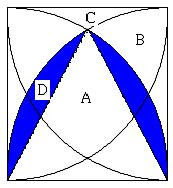2009年8月22日 星期六

The solution of quadrilaterals areas – part IA + 4B + 4C = 1 ..... (1)
A + 3B + 2C = π/ 4 ..... (2)
A + 2B = π/ 2 – 1 ..... (3)

A + 4B + 4C = 1 ..... (1)
A + 3B + 2C = π/ 4 ..... (2)
A / 2 + B = π/ 4 – 1 / 2 ..... (4)A + 4B + 4C = 1 ..... (1)
A + 3B + 2C = π / 4 ..... (2)
B + C + D = π / 12 ..... (5)
A + 2B + C –2D = √3 / 4 ..... (6)

A = 1 + π / 3 – √3